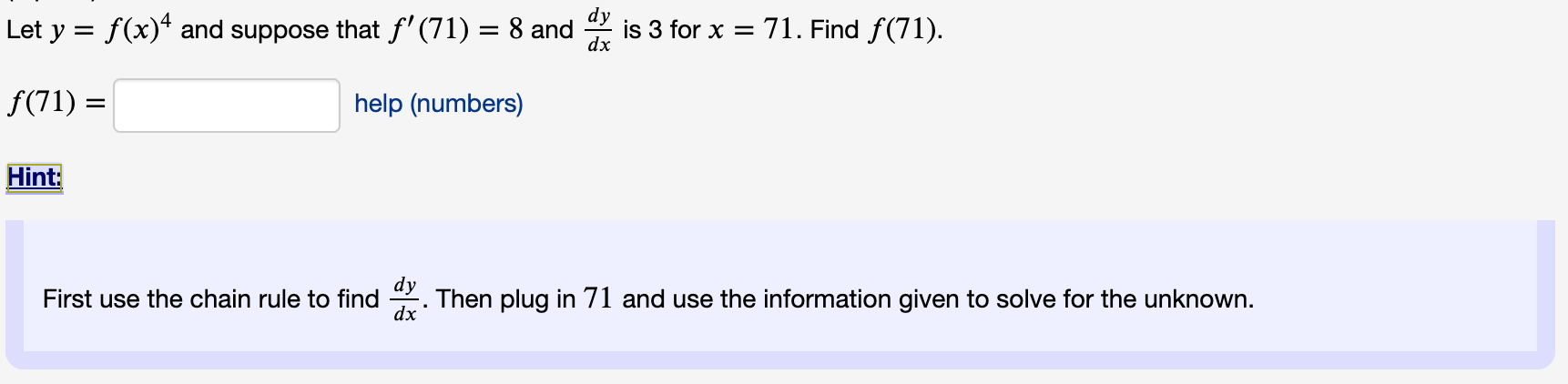# dy Let y = f(x)* and suppose that f'(71) = 8 and is 3 for x = 71. Find f(71). dx

Questionhelp_outlineImage Transcriptionclosedy Let y = f(x)* and suppose that f'(71) = 8 and is 3 for x = 71. Find f(71). dx fullscreen

### Want to see this answer and more?

Experts are waiting 24/7 to provide step-by-step solutions in as fast as 30 minutes!*

*Response times may vary by subject and question complexity. Median response time is 34 minutes for paid subscribers and may be longer for promotional offers.
Tagged in
Math
Calculus

### Derivative